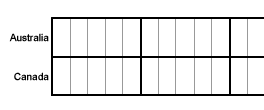# Data and Graphs

Our Data and Graphs unit shows students how to read and interpret line, bar and circle graphs. Students are also shown how to construct these graphs from data in a table. They will then learn how to determine which graphs are appropriate for a given set of data. Try our sample lessons below, or browse other units.Data and Graphs Description Data and Line Graphs Students are introduced to the parts of a line graph and the purpose of each. They are shown how to read and interpret data from a line graph. Constructing Line Graphs Students are shown how to construct a line graph from a set of data. Step-by-step procedures are listed along with large, detailed graphs for visual reference. Data and Bar Graphs Students are introduced to the parts of a bar graph and the purpose of each. They are then shown how to read and interpret data from horizontal and vertical graphs. Constructing Bar Graphs Students are shown how to construct a bar graph from a set of data. Step-by-step procedures are listed along with large, detailed bar graphs. Data and Circle Graphs Students are introduced to the parts of a circle graph and the purpose of each. They are shown how to read and interpret data from a circle graph. Constructing Circle Graphs Students are shown how to construct a circle graph from a set of data. They are also shown how to compute the angle measure for each item in the circle graph. Step-by-step procedures are listed along with large, detailed graphs. Comparing Graphs Students are presented with the purpose of each type of graph. They are then shown how to determine which graph is appropriate for displaying a given set of data. Practice Exercises To complete 10 additional exercises as practice. To assess students' understanding of all data and graph concepts learned so far. Challenge Exercises To solve 10 additional problems that challenge students' understanding of data and graph concepts. To hone students' problem-solving skills. Solutions To review complete solutions to all exercises presented in this unit. Includes the problem, step-by-step solutions, final answer for each exercise. Learning Objectives Learn about Data and Line Graphs; Constructing Line Graphs; Data and Bar Graphs; Constructing Bar Graphs; Data and Circle Graphs; Constructing Circle Graphs; Comparing Graphs